Test: Constraints And Statical Determinacy

# Test: Constraints And Statical Determinacy

Test Description

## 15 Questions MCQ Test Engineering Mechanics | Test: Constraints And Statical Determinacy

Test: Constraints And Statical Determinacy for Mechanical Engineering 2023 is part of Engineering Mechanics preparation. The Test: Constraints And Statical Determinacy questions and answers have been prepared according to the Mechanical Engineering exam syllabus.The Test: Constraints And Statical Determinacy MCQs are made for Mechanical Engineering 2023 Exam. Find important definitions, questions, notes, meanings, examples, exercises, MCQs and online tests for Test: Constraints And Statical Determinacy below.
Solutions of Test: Constraints And Statical Determinacy questions in English are available as part of our Engineering Mechanics for Mechanical Engineering & Test: Constraints And Statical Determinacy solutions in Hindi for Engineering Mechanics course. Download more important topics, notes, lectures and mock test series for Mechanical Engineering Exam by signing up for free. Attempt Test: Constraints And Statical Determinacy | 15 questions in 30 minutes | Mock test for Mechanical Engineering preparation | Free important questions MCQ to study Engineering Mechanics for Mechanical Engineering Exam | Download free PDF with solutions
 1 Crore+ students have signed up on EduRev. Have you?
Test: Constraints And Statical Determinacy - Question 1

### To satisfy the conditions of the equilibrium, the must not only be having a net force zero, but also be properly held or constrained on its supports.

Detailed Solution for Test: Constraints And Statical Determinacy - Question 1

To satisfy the conditions of the equilibrium, the must not only be having a net force zero, but also be properly held or constrained on its supports. The more the stable are the supports the more the stable is the equilibrium, that is the more easily is the equilibrium is achived.

Test: Constraints And Statical Determinacy - Question 2

### A homogeneous plate is shown has a mass of 100kg and is subjected to a force and a system of couple along its edge. If it is supported by a horizontal plane and a roller at R a ball and socket joint at P and a cord at Q, determine the vertical reaction at the support P.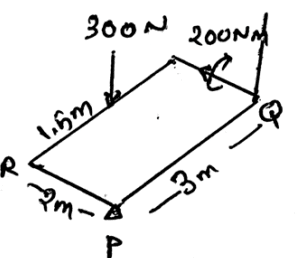Detailed Solution for Test: Constraints And Statical Determinacy - Question 2

As the vertical force means that the force is going from the outer to the inner section of the plate and the horizontal force means that the force is travelling along the plane of the surface. Thus by applying the conditions for the equilibrium we get the above answer.

Test: Constraints And Statical Determinacy - Question 3

### What is redundant support?

Detailed Solution for Test: Constraints And Statical Determinacy - Question 3

The supports if are more than the supports required to hold the structure are termed as the reductant supports. It becomes statically indeterminate. This not only creates a problem in achieving the equilibrium but also affects the calculation of the equilibrium.

Test: Constraints And Statical Determinacy - Question 4

A homogeneous plate is shown has a mass of 100kg and is subjected to a force and a system of couple along its edge. If it is supported by a horizontal plane and a roller at R a ball and socket joint at P and a cord at Q, determine the horizontal reaction at the support P.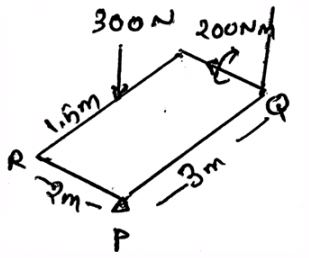Detailed Solution for Test: Constraints And Statical Determinacy - Question 4

As the vertical force means that the force is going from the outer to the inner section of the plate and the horizontal force means that the force is travelling along the plane of the surface. Thus by applying the conditions for the equilibrium we get the above answer.

Test: Constraints And Statical Determinacy - Question 5

Statically indeterminacy means?

Detailed Solution for Test: Constraints And Statical Determinacy - Question 5

Statically indeterminacy is the condition when less equations are available for equilibrium than the unknown loadings. This not only creates a problem in achieving the equilibrium but also affects the calculation of the equilibrium. Thus this is the stuff which one needs to prevent from happening.

Test: Constraints And Statical Determinacy - Question 6

A homogeneous plate is shown has a mass of 100kg and is subjected to a force and a system of couple along its edge. If it is supported by a horizontal plane and a roller at R a ball and socket joint at P and a cord at Q, determine the horizontal reaction at the support R.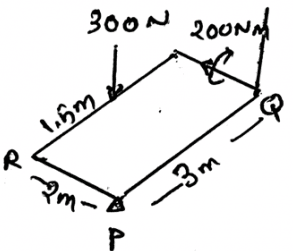Detailed Solution for Test: Constraints And Statical Determinacy - Question 6

As the vertical force means that the force is going from the outer to the inner section of the plate and the horizontal force means that the force is travelling along the plane of the surface. Thus by applying the conditions for the equilibrium we get the above answer.

Test: Constraints And Statical Determinacy - Question 7

The additional equations invented in the statically indeterminacy condition is solved by __________

Detailed Solution for Test: Constraints And Statical Determinacy - Question 7

The additional equations invented in the statically indeterminacy condition is solved by deformation conditions at the point of supports. This are the conditions which are based on the physical and the chemical properties of the body. A different study for this is done in mechanics of material.

Test: Constraints And Statical Determinacy - Question 8

The generation of equal equations available for equilibrium as the unknown loadings always guarantees the stability of the body.

Detailed Solution for Test: Constraints And Statical Determinacy - Question 8

The generation of equal equations available for equilibrium as the unknown loadings doesn’t always guarantees the stability of the body. It is defined on the basis of the supports that are their supporting the body. Or it also depends on the types of the forces that are acting, i.e. the three or two force system.

Test: Constraints And Statical Determinacy - Question 9

In 3D the body will be improperly constrained if the axis is intersected by the reaction forces.

Detailed Solution for Test: Constraints And Statical Determinacy - Question 9

In 3D the body will be improperly constrained if the axis is intersected by the reaction forces. This is because there will be small moments which will occur in the real situations. But they will not be matched in the calculations done for determining the equilibrium. Thus the statement is right.

Test: Constraints And Statical Determinacy - Question 10

The improper constraining leading to instability occurs when ___________________

Detailed Solution for Test: Constraints And Statical Determinacy - Question 10

If the forces are parallel (the reactive forces) then this creates an instability. That is this is also a type of improper constraining which is resulting in instability of the structure. Thus reactive forces are parallel are resulting in the instability of the body.

Test: Constraints And Statical Determinacy - Question 11

A homogeneous plate is shown has a mass of 100kg and is subjected to a force and a system of couple along its edge. If it is supported by a horizontal plane and a roller at R a ball and socket joint at P and a cord at Q, determine the sum of all the vertical forces at the supports.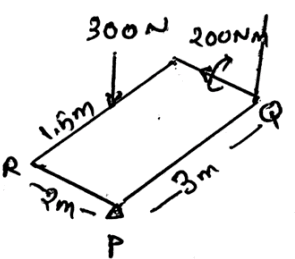Detailed Solution for Test: Constraints And Statical Determinacy - Question 11

As the vertical force means that the force is going from the outer to the inner section of the plate and the horizontal force means that the force is travelling along the plane of the surface. Thus by applying the conditions for the equilibrium we get the above answer.

Test: Constraints And Statical Determinacy - Question 12

A homogeneous plate is shown has a mass of 100kg and is subjected to a force and a system of couple along its edge. If it is supported by a horizontal plane and a roller at R a ball and socket joint at P and a cord at Q, determine the vertical reaction at the support Q.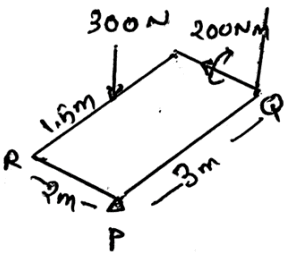Detailed Solution for Test: Constraints And Statical Determinacy - Question 12

As the vertical force means that the force is going from the outer to the inner section of the plate and the horizontal force means that the force is travelling along the plane of the surface. Thus by applying the conditions for the equilibrium we get the above answer.

Test: Constraints And Statical Determinacy - Question 13

What is partially constrained?

Detailed Solution for Test: Constraints And Statical Determinacy - Question 13

The situation may arise when there will be more equations available for equilibrium than the reactive forces. This condition is known as partially constrained. This is also one of the factor which is giving instability to the structure.

Test: Constraints And Statical Determinacy - Question 14

If all the reactive forces are intersecting at the common point or pass through a common axis when _____________

Detailed Solution for Test: Constraints And Statical Determinacy - Question 14

If all the reactive forces are intersecting at the common point or pass through a common axis then the body is said to be improperly constrained. Whether it may be the reactive forces being parallel. Or be it the forces meeting at a common point. The body is said to be improperly constrained.

Test: Constraints And Statical Determinacy - Question 15

A homogeneous plate is shown has a mass of 100kg and is subjected to a force and a system of couple along its edge. If it is supported by a horizontal plane and a roller at R a ball and socket joint at P and a cord at Q, determine the vertical reaction at the support R.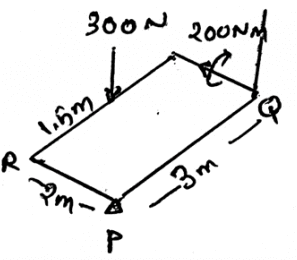Detailed Solution for Test: Constraints And Statical Determinacy - Question 15

As the vertical force means that the force is going from the outer to the inner section of the plate and the horizontal force means that the force is travelling along the plane of the surface. Thus by applying the conditions for the equilibrium we get the above answer.

## Engineering Mechanics

24 videos|71 docs|58 tests
 Use Code STAYHOME200 and get INR 200 additional OFF Use Coupon Code
Information about Test: Constraints And Statical Determinacy Page
In this test you can find the Exam questions for Test: Constraints And Statical Determinacy solved & explained in the simplest way possible. Besides giving Questions and answers for Test: Constraints And Statical Determinacy, EduRev gives you an ample number of Online tests for practice

## Engineering Mechanics

24 videos|71 docs|58 tests